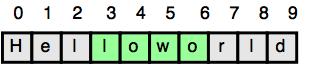## Algorithm

Problem Name: Java Substring

In this HackerRank Functions in Java programming problem solution,

iven a string, , and two indices, start and end, print a substring consisting of all characters in the inclusive range from start to end - 1.You'll find the String class' substring method helpful in completing this challenge.

Input Format

The first line contains a single string denoting s .

The second line contains two space-separated integers denoting the respective values of start and end.

Constraints

• 1 <= |s| <= 100
• 0 <= start <= end <= n
• String s consists of English alphabetic letters (i.e., [a - zA - Z]) only.

Output Format

Print the substring in the inclusive range from start to end - 1

Sample Input

Helloworld
3 7


Sample Output

lowo


Explanation

In the diagram below, the substring is highlighted in green:## Code Examples

### #1 Code Example with Java Programming

Code - Java Programming


import java.util.Scanner;

public class Solution{
public static void main(String[] args){
Scanner input = new Scanner(System.in);

String s = input.next();
int a = input.nextInt();
int b = input.nextInt();

System.out.println(s.substring(a, b));
}
}

Copy The Code &